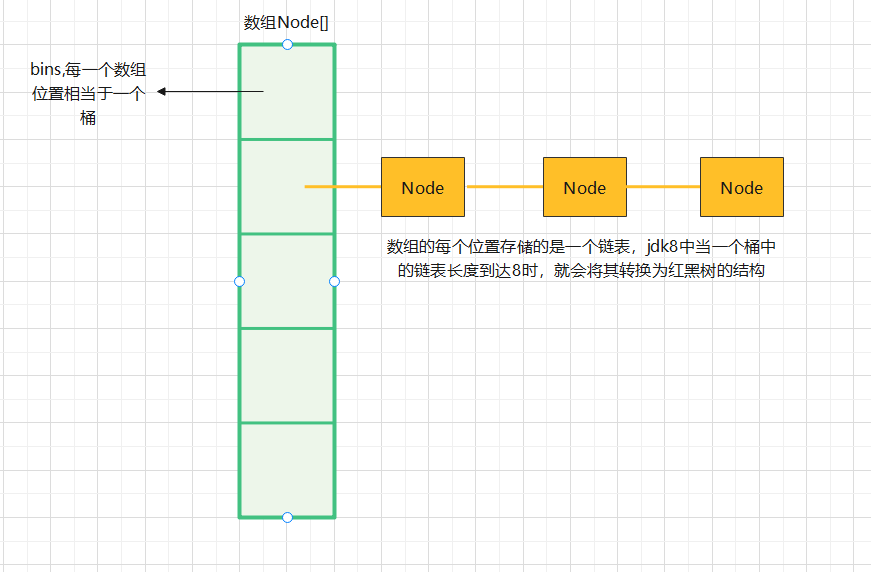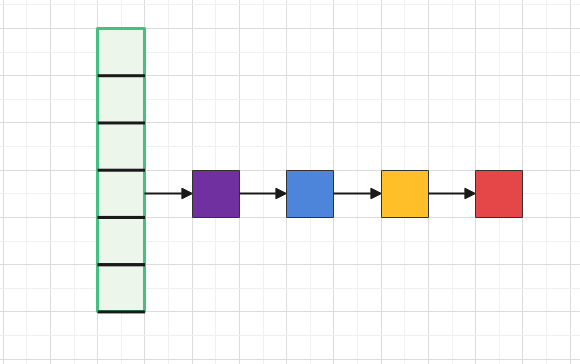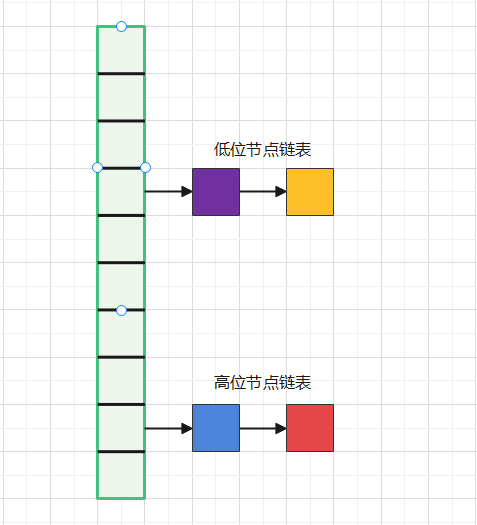# HashMap源码解析和设计解读

## HashMap源码解析

​ 想要理解HashMap底层数据的存储形式，底层原理，最好的形式就是读它的源码，但是说实话，源码的注释说明全是英文，英文不是非常好的朋友读起来真的非常吃力，我基本上看了差不多七八遍，还结合网上的一些解析，才觉得自己有点理解。

​ 我先画了一个图，HashMap数据存储的结构图，先有个理解，再来看看下面的代码解析可能会好理解些。

HashMap的数据结构### HashMap静态属性


/**
* The default initial capacity - MUST be a power of two.
* 默认的数组容量16
*/
static final int DEFAULT_INITIAL_CAPACITY = 1 << 4; // aka 16

/**
* 最大的容量
*/
static final int MAXIMUM_CAPACITY = 1 << 30;

/**
* The load factor used when none specified in constructor.
* 负载因子，用于扩容，当数组的容量大于等于 0.75*DEFAULT_INITIAL_CAPACITY时，就要扩容
*/
static final float DEFAULT_LOAD_FACTOR = 0.75f;

/**
* 每个桶中数据结构转变为树的链表长度界限，当链表长度为为8时，转成红黑树
*/
static final int TREEIFY_THRESHOLD = 8;

/**
* 当树的结点等于小于等于6时，又转会链表
*/
static final int UNTREEIFY_THRESHOLD = 6;

static final int MIN_TREEIFY_CAPACITY = 64;




/**
* Basic hash bin node, used for most entries.  (See below for
* TreeNode subclass, and in LinkedHashMap for its Entry subclass.)
*/
static class Node<K,V> implements Map.Entry<K,V> {
final int hash;
final K key;
V value;
Node<K,V> next;

Node(int hash, K key, V value, Node<K,V> next) {
this.hash = hash;
this.key = key;
this.value = value;
this.next = next;
}

……省略
}



/**
* 这就是HashMap存储数据的本质容器，数组，只不过是Node数组，里面存储的是
* Node节点
*/
transient Node<K,V>[] table;

/**
* Holds cached entrySet(). Note that AbstractMap fields are used
* for keySet() and values().
*/
transient Set<Map.Entry<K,V>> entrySet;

/**
* The number of key-value mappings contained in this map.
*/
transient int size;

/**
* 这是用来标记HashMap结构变化的次数的
*/
transient int modCount;


### 如何存放数据

public V put(K key, V value) {
return putVal(hash(key), key, value, false, true);
}


static final int hash(Object key) {
int h;
return (key == null) ? 0 : (h = key.hashCode()) ^ (h >>> 16);
}
// 这 hashCode是一个本地方法，不管，那为什么又要和无符号右移16位做异或运算呢？

final V putVal(int hash, K key, V value, boolean onlyIfAbsent,
boolean evict) {
// tab是用来操作存储容器的，p是存储的节点，n是数组的长度，i是数组位置下标
Node<K,V>[] tab; Node<K,V> p; int n, i;
if ((tab = table) == null || (n = tab.length) == 0)
// 数组容器初始化resize(),这个方法后面重点看，就是重新设置容量大小
n = (tab = resize()).length;
// 如果找到的这个位置的Node节点为null，就直接new一个，将put的数据存放进来
// (table.length-1)&hash 是HashMap中确定数组存放位置的方式
if ((p = tab[i = (n - 1) & hash]) == null)
tab[i] = newNode(hash, key, value, null);
else {
// 进到这里，就说明出现了hash碰撞，生成的hash值一样了，找到的位置已经有值了，p不为null
// e是一个临时节点变量
Node<K,V> e; K k;
// 如果hash值一样，key也一样，那就是覆盖嘛，直接吧p给e，到下面或进行value的新旧替换
if (p.hash == hash &&
((k = p.key) == key || (key != null && key.equals(k))))
e = p;
// 如果key不一样，判断是否是树节点了，就用树的增加节点方法，这里我们先不研究
else if (p instanceof TreeNode)
e = ((TreeNode<K,V>)p).putTreeVal(this, tab, hash, key, value);
else {
// 如果还不是树节点，就遍历这个桶的链表，将数据加到这个链表的最后
for (int binCount = 0; ; ++binCount) {
if ((e = p.next) == null) {
p.next = newNode(hash, key, value, null);
// 如果已经大于等于7了，就转成红黑树
if (binCount >= TREEIFY_THRESHOLD - 1) // -1 for 1st
treeifyBin(tab, hash);
break;
}
if (e.hash == hash &&
((k = e.key) == key || (key != null && key.equals(k))))
break;
p = e;
}
}
// 到这里就是key相同的时候，新旧值替换
if (e != null) { // existing mapping for key
V oldValue = e.value;
if (!onlyIfAbsent || oldValue == null)
e.value = value;
return oldValue;
}
}
++modCount; // 修改记录，这个只有当数组新增了值才会到这里，想上面只增加再同一个桶中的链表后，不会加一
if (++size > threshold)
resize(); // 扩容
afterNodeInsertion(evict);
return null;
}


putVal方法整体概括下逻辑应该分为以下几点：

• 首先先根据key得到hashCode值，确认存放的数组位置
• 该位置如果没有值，就直接new一个Node节点存放进去
• 该位置有值，又分为两种情况
• 如果key相同，则就是替换嘛，把这个key所对应的value给换成新的
• 如果key不相同，则就是hash冲突，就需要增加该桶的链表长度了，将该数据增加到该链表的后边。

### 扩容机制

final Node<K,V>[] resize() {
// 定义一个Node[]
Node<K,V>[] oldTab = table;
// 老的容量
int oldCap = (oldTab == null) ? 0 : oldTab.length;
// 老的阈值
int oldThr = threshold;
// 新容量，新阈值
int newCap, newThr = 0;
if (oldCap > 0) {
if (oldCap >= MAXIMUM_CAPACITY) {
threshold = Integer.MAX_VALUE;
return oldTab;
}
// 每次扩容都是前一次的两倍，阈值也是
else if ((newCap = oldCap << 1) < MAXIMUM_CAPACITY &&
oldCap >= DEFAULT_INITIAL_CAPACITY)
newThr = oldThr << 1; // double threshold
}
else if (oldThr > 0) // initial capacity was placed in threshold
newCap = oldThr;
else {               // zero initial threshold signifies using defaults
// 这里是最开是初始化的时候，默认容量是16，默认的阈值时0.75*16=12
newCap = DEFAULT_INITIAL_CAPACITY;
}
if (newThr == 0) {
float ft = (float)newCap * loadFactor;
newThr = (newCap < MAXIMUM_CAPACITY && ft < (float)MAXIMUM_CAPACITY ?
(int)ft : Integer.MAX_VALUE);
}
threshold = newThr;

……#############这下面才是扩容机制中重新确定每个Node节点所在的位置的精髓所在，单独讲#################
}


static final int hash(Object key) {
int h;
return (key == null) ? 0 : (h = key.hashCode()) ^ (h >>> 16);
}

1. 首先需要确定的一点是，用hash值是为了快速检索定位，但是需要好的hash算法去减少hash冲突，提高离散性。以这点作为基础，猜想上面这样算法是因为降低hash冲突，提高离散性。
2. (table.length-1) & hash还是再看确定bin下表的方式，table的长度和hash值做与运算，在实际中table的长度并不会特别大，2的16次方都比较少，更何况是32位，所以如果直接用hashCode()方法生成的hash值做运算，其实大概率只用到了后16位，前十六位就浪费了。所以(h = key.hashCode()) ^ (h >>> 16)先用右移16位做异或运算，其实就是后16位和前16位做异或运算，这样在确定下标中hash的32位都参与了运算，这样既就增加了随机性，提高了离散性。
3. 为什么是做"异或"运算呢？因为相比于“与”运算和“或”运算，“异或”运算生成0和1的概率是相等的，没有偏重哪一个。
@SuppressWarnings({"rawtypes","unchecked"})
// 确定完容量后，new一个新的数组，将新的数组赋给table这个数组
Node<K,V>[] newTab = (Node<K,V>[])new Node[newCap];
table = newTab;
if (oldTab != null) {
// 这里就是来确定老的table中每个桶中每个Node在新的table中的位置是否要移动，怎么移动
// 这里如果不明白为什么需要在新表中重新确定位置的，看下面的图解可能好理解
for (int j = 0; j < oldCap; ++j) {
// 临时节点e
Node<K,V> e;
// 取出第j个位置的节点给e
if ((e = oldTab[j]) != null) {
oldTab[j] = null;
// 这说明在这个原来的j位置上不存在hash碰撞，就直接放到新table中相同的位置就行
if (e.next == null)
newTab[e.hash & (newCap - 1)] = e;
else if (e instanceof TreeNode)
((TreeNode<K,V>)e).split(this, newTab, j, oldCap);
else { // preserve order
// 进到这个else就说明在原来j位置存在hash碰撞，形成链表了，e.next不为null
Node<K,V> loHead = null, loTail = null;
// 高位节点
Node<K,V> hiHead = null, hiTail = null;
Node<K,V> next;
// 循环遍历这个桶的链表的每个节点，重新弄确定位置
do {
next = e.next;
// 这个条件的&运算非常巧妙，如果为0，就说明这个节点不需要移动位置，在新table中也是j这个位置的桶中
// 这里这个&运算的结果不为0就为1，下面详细介绍
if ((e.hash & oldCap) == 0) {
// 这里是理清节点的关系，相当于这里是这个桶中所有不需要移位的Node,又要形成一个新的链表
if (loTail == null)
else
loTail.next = e;
loTail = e;
}
else {
// 那这就当然就是需要移位的节点链表了咯
if (hiTail == null)
else
hiTail.next = e;
hiTail = e;
}
} while ((e = next) != null);
if (loTail != null) {
loTail.next = null;
// 这里就是低位节点，不需要移位，在新的table中还是j位置
}
if (hiTail != null) {
hiTail.next = null;
// 高位节点在新的table中的位置就变成 （原来的位置+原来的容量）
}
}
}
}
}
return newTab;### 非线程安全

jdk1.8中的HashMap线程不安全主要是在多线程并发的时候出现数据覆盖的情况，在putVal方法中

final V putVal(int hash, K key, V value, boolean onlyIfAbsent,
boolean evict) {
// tab是用来操作存储容器的，p是存储的节点，n是数组的长度，i是数组位置下标
Node<K,V>[] tab; Node<K,V> p; int n, i;
if ((tab = table) == null || (n = tab.length) == 0)
// 数组容器初始化resize(),这个方法后面重点看，就是重新设置容量大小
n = (tab = resize()).length;
// 如果找到的这个位置的Node节点为null，就直接new一个，将put的数据存放进来
// (table.length-1)&hash 是HashMap中确定数组存放位置的方式
if ((p = tab[i = (n - 1) & hash]) == null)
tab[i] = newNode(hash, key, value, null);


posted @ 2021-06-14 18:01  红细胞司令  阅读(199)  评论(1编辑  收藏  举报